SSC (Marathi Semi-English) 10thMaharashtra State Board
Share
Notifications

View all notifications
Books Shortlist
Your shortlist is empty

# Question Paper Solutions for Geometry Balbharati Model Question Paper Set 2 2018-2019 SSC (Marathi Semi-English) 10th

Login
Create free account

Forgot password?
Geometry
Balbharati Model Question Paper Set 2
2018-2019 March
Marks: 40

1
1.A | Solve any four of the following.
1.A.1

In the figure, line PQ || line RS. Using the information given
in the figure find the value of x.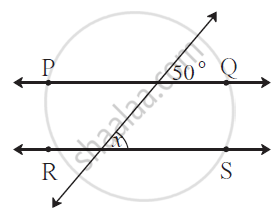Chapter:  Co-ordinate Geometry
Concept: General Equation of a Line
1.A.2

In the figure, parts of the two triangles bearing identical marks are
congruent. State the test by which the triangles are congruent.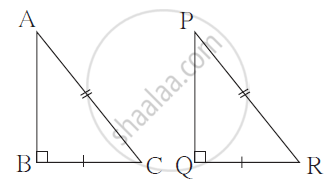Chapter:  Similarity
Concept: Similarity of Triangles
1.A.3

In Δ ABC, if ∠ A = 65° ; ∠ B = 40° then find the measure of ∠ C.

Chapter:  Mensuration
Concept: Length of an Arc
1.A.4

squarePQRS is a parallelogram. Write the sum of measures of ∠P and ∠ Q.

Chapter:  Circle
Concept: Property of Sum of Measures of Arcs
1.A.5

If hypotenuse of a right angled triangle is 5 cm, find the radius of
the circle passing through all vertices of the triangle.

Chapter:  Pythagoras Theorem
Concept: Apollonius Theorem
1.A.6

Write the co-ordinates of the point of intersection of graphs of
equations x = 2 and y = -3.

Chapter:  Co-ordinate Geometry
Concept: Co-ordinates of the Midpoint of a Segment
1.B | Solve any two of the following.
1.B.1

Length of a rectangular tank is twice its breadth. If the
depth of the tank is 3 m and area of its four walls is 108 m2, find the
length of the tank.

Chapter:  Mensuration
Concept: Length of an Arc
1.B.2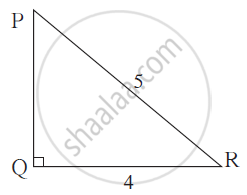In right angled triangle PQR,
if ∠ Q = 90°, PR = 5,
QR = 4 then find PQ and hence find tan R.

Chapter:  Pythagoras Theorem
Concept: Similarity in Right Angled Triangles
1.B.3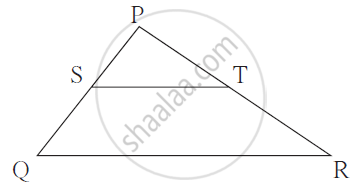In Δ PQR, points S and T
are the midpoints of sides PQ
and PR respectively.
If ST = 6.2 then find the length of QR.

Chapter:  Pythagoras Theorem
Concept: Application of Pythagoras Theorem in Acute Angle and Obtuse AngleIn Δ PQR, points S and T
are the midpoints of sides PQ
and PR respectively.
If ST = 6.2 then find the length of QR.

Chapter:  Similarity
Concept: Application of Pythagoras Theorem in Acute Angle and Obtuse Angle
2
2.A | Select the appropriate alternative and write it.
2.A.1

Δ ABC ∼ Δ PQR. If A(Δ ABC)=25, A(ΔPQR)=16, find AB : PQ.
(A) 25:16
(B) 4:5
(C) 16:25
(D) 5:4

Chapter:  Similarity
Concept: Similar Triangles
2.A.2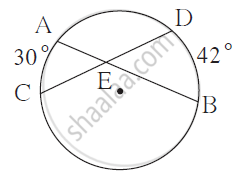From the information given in the figure, find the measure of ∠ AEC.
(A) 42° (B) 30°
(C) 36° (D) 72°

Chapter:  Circle
Concept: Property of Sum of Measures of Arcs
2.A.3

Point P is the midpoint of seg AB. If co-ordinates of A and B are (-4, 2) and (6, 2) respectively then find the co-ordinates of point P.
(A) (-1,2) (B) (1,2) (C) (1,-2) (D) (-1,-2)

Chapter:  Co-ordinate Geometry
Concept: Co-ordinates of the Midpoint of a Segment
2.A.4

Find the ratio of the volumes of a cylinder and a cone having equal radius and equal height.
(A)1 : 2 (B) 2 : 1 (C) 1 : 3 (D) 3 : 1

Chapter:  Mensuration
Concept: Introduction of Surface Areas and Volumes
2.B | Solve any two of the following.
2.B.1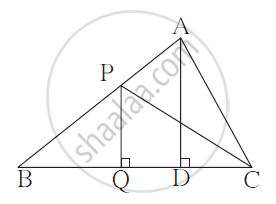In the adjoining figure,
PQ ⊥ BC, AD ⊥ BC,
PQ = 4, AD = 6
Write down the following ratios.
(i)(A(ΔPQB))/(A(ΔADB))

(ii)(A(ΔPBC))/(A(ΔABC))

Chapter:  Similarity
Concept: Similar Triangles
2.B.2

Diagonal of a square is 20 cm. Find the length and perimeter of the square.

Chapter:  Mensuration
Concept: Perimeter and Area of a Circle
2.B.3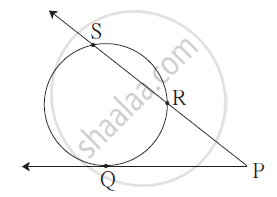In the figure, point Q is the
point of contact. If PQ = 12,
PR = 8 then find PS.

Chapter:  Circle
Concept: Tangent to a Circle
3
3.A | Carry out any two activities of the following.
3.A.1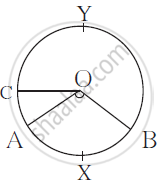In the following figure ‘O’ is the centre of the circle.

∠AOB = 1100, m(arc AC) = 450.

Use the information and fill in the boxes with proper numbers.

(i) m(arcAXB) =

(ii)m(arcCAB) =
(iv)∠COB =

(iv)m(arcAYB) =

Chapter:  Circle
Concept: Tangent Segment Theorem
3.A.2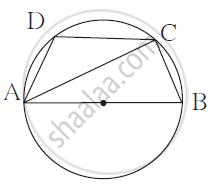In the figure, c ABCD is a cyclic quadrilateral. Seg AB is a diameter. If ∠ ADC = 120˚, complete the following activity to find measure of ∠ BAC.

square ABCD is a cyclic quadrilateral.
∴ ∠ ADC + ∠ ABC = 180°
∴ 120˚ + ∠ ABC = 180°
∴ ∠ ABC =
But ∠ ACB = .......angle in semicircle In Δ ABC,
∠ BAC + ∠ ACB + ∠ ABC = 180°
∴ ∠BAC + = 180°
∴ ∠ BAC =

Chapter:  Circle
Concept: Cyclic Properties
3.A.3

Complete the table below the graph with the help of the following graph.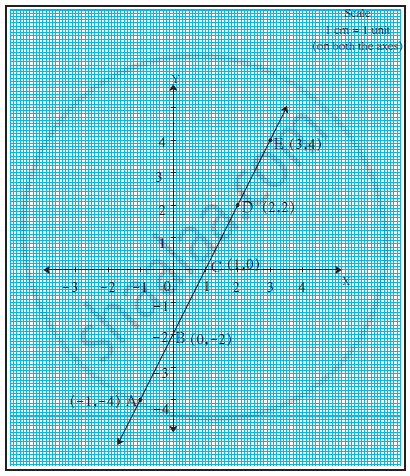Sr. No. First point Second point Co-ordinates of first point (x1 , y1) Co-ordinates of second point (x2 , y2) (y_2 - y_2)/(x_2 - x_2) 1 C E (1, 0) (3,4) 4/2=2 2 A B (-1,-4) (0,-2) 2/1 = 2 3 B D (0,-2) (2,2) 4/2=2
Chapter:  Co-ordinate Geometry
Concept: Co-ordinates of the Midpoint of a Segment
3.B | Solve any two of the following.
3.B.1

If tanθ = 3/4 then find the value of secθ.

Chapter:  Trigonometry
Concept: Trigonometric Identities
3.B.2

Find the length of an arc if measure of the arc is 90° and its radius
is 14 cm.

Chapter:  Mensuration
Concept: Length of an Arc
3.B.3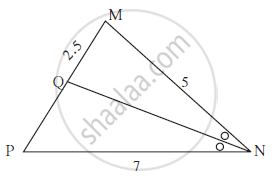Seg NQ is the bisector of ∠ N
of Δ MNP. If MN= 5, PN =7,
MQ = 2.5 then find QP.

Chapter:  Similarity
Concept: Property of an Angle Bisector of a Triangle
4 | Solve any three of the following.
4.A

∆ABC is an equilateral triangle. Point P is on base BC such that PC = $\frac{1}{3}$ BC, if AB = 6 cm find AP.

Chapter:  Pythagoras Theorem
Concept: Similarity in Right Angled Triangles
4.B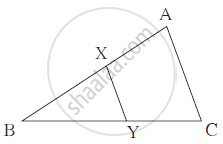In the adjoining figure,
seg XY || seg AC, If 3AX = 2BX
and XY = 9 then find the length of AC.

Chapter:  Similarity
Concept: Basic Proportionality Theorem Or Thales Theorem
4.C

Show that square ABCD formed by the vertices A(-4,-7), B(-1,2), C(8,5) and D(5,-4) is a rhombus.

Chapter:  Co-ordinate Geometry
Concept: Concepts of Coordinate Geometry
4.D

Two buildings are in front of each other on a road of width 15 meters. From the top of the first building, having a height of 12 meter, the angle of elevation of the top of the second building is 30°.What is the height of the second building?

Chapter:  Trigonometry
Concept: Heights and Distances
5 | Solve any one of the following.
5.A

Two circles intersect each other at points C and D. Their common tangent AB touches the circles at point A and B. Prove that :
∠ ADB + ∠ ACB = 180°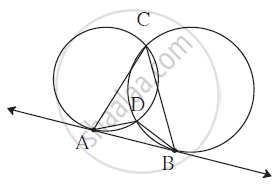Chapter:  Circle
Concept: Tangent Properties - If Two Circles Touch, the Point of Contact Lies on the Straight Line Joining Their Centers
5.B

Draw an isosceles triangle with base 5 cm and height 4 cm. Draw a triangle similar to the triangle drawn whose sides are 2/3 times the sides of the triangle.

Chapter:  Similarity
Concept: Basic Proportionality Theorem Or Thales Theorem
6 | Solve any one of the following:
6.A

Height of a cylindrical barrel is 50 cm and radius of its base is 20 cm. Anurag started to fill the barrel with water, when it was empty, by a cylindrical mug. The diameter and height of the mug was 10 cm and 15cm respectively. How many minium number of mugs will be required for the barrel to overflow?

Chapter:  Circle
Concept: Theorem - Converse of Tangent at Any Point to the Circle is Perpendicular to the Radius
6.B

Draw Δ ABC such that, AB = 8 cm, BC = 6 cm and ∠ B = 90°. Draw seg BD
perpendicular to hypotenuse AC. Draw a circle passing through points
B, D, A. Show that line CB is a tangent of the circle.

Chapter:  Circle
Concept: Tangent to a Circle

#### Request Question Paper

If you dont find a question paper, kindly write to us

View All Requests

#### Submit Question Paper

Help us maintain new question papers on shaalaa.com, so we can continue to help students

only jpg, png and pdf files

## Maharashtra State Board previous year question papers 10th Geometry with solutions 2018 - 2019

Maharashtra State Board 10th Geometry question paper solution is key to score more marks in final exams. Students who have used our past year paper solution have significantly improved in speed and boosted their confidence to solve any question in the examination. Our Maharashtra State Board 10th Geometry question paper 2019 serve as a catalyst to prepare for your Geometry board examination.
Previous year Question paper for Maharashtra State Board 10th Geometry-2019 is solved by experts. Solved question papers gives you the chance to check yourself after your mock test.
By referring the question paper Solutions for Geometry, you can scale your preparation level and work on your weak areas. It will also help the candidates in developing the time-management skills. Practice makes perfect, and there is no better way to practice than to attempt previous year question paper solutions of Maharashtra State Board 10th.

How Maharashtra State Board 10th Question Paper solutions Help Students ?
• Question paper solutions for Geometry will helps students to prepare for exam.
• Question paper with answer will boost students confidence in exam time and also give you an idea About the important questions and topics to be prepared for the board exam.
• For finding solution of question papers no need to refer so multiple sources like textbook or guides.
S